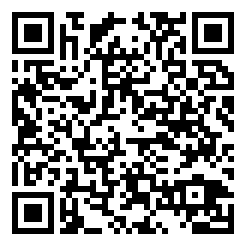OpenCV 图像遍历与颜色缩减Nightn 1月 21, 2017
• 在其它设备中阅读本文章
•## 2. 图像存储方式## 3. 颜色缩减//color1输入,color2输出
color2 = (color1 / 23) * 23;


// 生成颜色查找表
vector<int> color_table;
int width = 20;
for (int i = 0; i < 256; i++)
{
color_table.push_back(i / width * width);
}


## 4. 图像遍历

double time_begin = (double)getTickCount();
// do something
double time_end = (double)getTickCount();
double time = (time_end - time_begin) / getTickFrequency();


### 4.1 利用指针遍历

Mat& ScanImageAndReduceC(Mat& I, vector<int> color_table)
{
//只接收字符型矩阵
CV_Assert(I.depth() != sizeof(uchar));
int channels = I.channels(); //获取图像通道数
int row = I.rows * channels;
int col = I.cols;
uchar* p;
if (I.isContinuous()) //判断像素是否连续存储
{
col *= row;
row = 1;
}
for (int i = 0; i < row; i++)
{
p = I.ptr<uchar>(i);
for (int j = 0; j < col; j++)
{
p[j] = color_table[p[j]];
}
}
return I;
}### 4.2 利用迭代器遍历

Mat& ScanImageAndReduceIterator(Mat& I, vector<int> color_table)
{
//只接收字符型矩阵
CV_Assert(I.depth() != sizeof(uchar));
int channels = I.channels();
switch (channels)
{
case 1:
{
MatIterator_<uchar> it, end;
for (it = I.begin<uchar>(), end = I.end<uchar>(); it != end; it++)
*it = color_table[*it];
break;
}
case 3:
{
MatIterator_<Vec3b> it, end;
for (it = I.begin<Vec3b>(), end = I.end<Vec3b>(); it != end; it++)
{
(*it) = color_table[(*it)];
(*it) = color_table[(*it)];
(*it) = color_table[(*it)];
}
break;
}
}
return I;
}


### 4.3 通过相关返回值的 On-the-fly 地址遍历

Mat& ScanImageAndReduceRadomAccess(Mat& I, vector<int> color_table)
{
//只接收字符型矩阵
CV_Assert(I.depth() != sizeof(uchar));
int channels = I.channels();
switch (channels)
{
case 1:
{
for (int i = 0; i < I.rows; i++)
{
for (int j = 0; j < I.cols; j++)
{
I.at<uchar>(i, j) = color_table[I.at<uchar>(i, j)];
}
}
break;
}
case 3:
{
for (int i = 0; i < I.rows; i++)
{
for (int j = 0; j < I.cols; j++)
{
I.at<Vec3b>(i, j) = color_table[I.at<Vec3b>(i, j)];
I.at<Vec3b>(i, j) = color_table[I.at<Vec3b>(i, j)];
I.at<Vec3b>(i, j) = color_table[I.at<Vec3b>(i, j)];
}
}
break;
}
}
return I;
}


### 4.4 核心函数 LUT (The Core Function)

Mat table(1, 256, CV_8U);
uchar* p = table.data;
for(int i = 0; i < 256; i++)
p[i] = color_table[i];


//image是输入，image_reduce是输出
LUT(image, table, image_reduce);


More